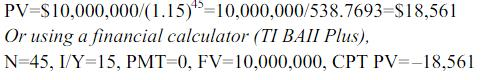Courses

# Test: Time Value Of Money

## 10 Questions MCQ Test Business Mathematics and Logical Reasoning & Statistics | Test: Time Value Of Money

Description
This mock test of Test: Time Value Of Money for CA Foundation helps you for every CA Foundation entrance exam. This contains 10 Multiple Choice Questions for CA Foundation Test: Time Value Of Money (mcq) to study with solutions a complete question bank. The solved questions answers in this Test: Time Value Of Money quiz give you a good mix of easy questions and tough questions. CA Foundation students definitely take this Test: Time Value Of Money exercise for a better result in the exam. You can find other Test: Time Value Of Money extra questions, long questions & short questions for CA Foundation on EduRev as well by searching above.
QUESTION: 1

Solution:
QUESTION: 2

Solution:
QUESTION: 3

### Suppose an investor wants to have \$10 million to retire 45 years from now. How much would she have to invest today with an annual rate of return equal to 15 percent?

Solution:QUESTION: 4

Which of the following is false?

Solution:
QUESTION: 5

Maggie deposits \$10,000 today and is promised a return of \$17,000 in eight years. What is the implied annual rate of return?

Solution:
QUESTION: 6

To triple \$1 million, Mika invested today at an annual rate of return of 9 percent. How long will it take Mika to achieve his goal?

Solution:

FV=PV(1+k)n
(3)(1,000,000)=1,000,000(1.09) n
ln(3)=(n)ln(1.09)
n=12.7 years
Or using a financial calculator (TI BAII Plus),
I/Y=9, PV= –1,000,000, PMT=0, FV=3,000,000, CPT N=12.7 years

option "C"

QUESTION: 7

Which of the following concepts is incorrect?

Solution:
QUESTION: 8

Jan plans to invest an equal amount of \$2,000 in an equity fund every year-end beginning this year. The expected annual return on the fund is 15 percent. She plans to invest for 20 years. How much could she expect to have at the end of 20 years?

Solution:
QUESTION: 9

In Problem 8, what is the present value of Jan’s investments?

Solution:
QUESTION: 10

What is the present value of a perpetuity with an annual year-end payment of \$1,500 and expected annual rate of return equal to 12 percent?

Solution: